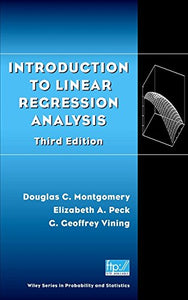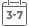# Introduction To Linear Regression Analysis, 3Rd Edition

• Publish Date: 2001-04-02
• Binding: Hardcover
• Author: Douglas C. Montgomery;Elizabeth A. Peck;G. Geoffrey Vining
Vendor
Wiley-Interscience
Regular price
\$52.26
Sale price
\$33.14
Quantity must be 1 or more

Attention: For textbook, access codes and supplements are not guaranteed with used items.Arrives in 3-7 Business Days

A comprehensive and thoroughly up-to-date look at regression analysis-still the most widely used technique in statistics today
As basic to statistics as the Pythagorean theorem is to geometry, regression analysis is a statistical technique for investigating and modeling the relationship between variables. With far-reaching applications in almost every field, regression analysis is used in engineering, the physical and chemical sciences, economics, management, life and biological sciences, and the social sciences.
Clearly balancing theory with applications, Introduction to Linear Regression Analysis describes conventional uses of the technique, as well as less common ones, placing linear regression in the practical context of today's mathematical and scientific research. Beginning with a general introduction to regression modeling, including typical applications, the book then outlines a host of technical tools that form the linear regression analytical arsenal, including: basic inference procedures and introductory aspects of model adequacy checking; how transformations and weighted least squares can be used to resolve problems of model inadequacy; how to deal with influential observations; and polynomial regression models and their variations. Succeeding chapters include detailed coverage of:
* Indicator variables, making the connection between regression and analysis-of-variance modelss
* Variable selection and model-building techniques
* The multicollinearity problem, including its sources, harmful effects, diagnostics, and remedial measures
* Robust regression techniques, including M-estimators, Least Median of Squares, and S-estimation
* Generalized linear models
The book also includes material on regression models with autocorrelated errors, bootstrapping regression estimates, classification and regression trees, and regression model validation. Topics not usually found in a linear regression textbook, such as nonlinear regression and generalized linear models, yet critical to engineering students and professionals, have also been included. The new critical role of the computer in regression analysis is reflected in the book's expanded discussion of regression diagnostics, where major analytical procedures now available in contemporary software packages, such as SAS, Minitab, and S-Plus, are detailed. The Appendix now includes ample background material on the theory of linear models underlying regression analysis. Data sets from the book, extensive problem solutions, and software hints are available on the ftp site. For other Wiley books by Doug Montgomery, visit our website at www.wiley.com/college/montgomery.